# Are two wrong models better than one? Missing data style

Would imputing + fitting an outcome model using the wrong variables be better than just fitting the wrong outcome model? Let’s investigate!
rstats
simulations
missing data
Author

Lucy D’Agostino McGowan

Published

April 29, 2023

After my previous post about missing data, Kathy asked on Twitter whether two wrong models (the imputation model + the outcome model) would be better than one (the outcome model alone).

Without doing any of the math, I’d guess the assumption of correctly spec the model also has a bigger impact in the CC analysis.

You need correct spec in MI, twice, but trade off that potential bias for higher prec.

This is a great question! I am going to investigate via a small simulation (so the answer could be “it depends”, but at least we will know how it seems to work in this very simple case) 😆.

Ok so here I have some predictor, `x` that is missing 50% of the time, dependent on `c_x` and `c_y`. The right imputation model would have `c_x`, the right outcome model needs `c_y`. Unfortunately, we only have access to one, which we will try to use in our imputation model (and outcome model). Let’s see whether two (wrong) models are better than one!

A “correct” model will be one that estimates that the coefficient for `x` is 1.

``````library(tidyverse)
library(broom)
library(mice)

n <- 1000

set.seed(928)

data <- tibble(
c_x = rnorm(n, sd = 0.71),
x = c_x + rnorm(n, sd = 0.71),
c_y = rnorm(n),
y = x + c_y + rnorm(n),
noise = rnorm(n),
x_miss = rbinom(n, 1, 1 / (1 + exp(-(c_x + c_y)))),
x_obs = ifelse(
x_miss,
NA,
x
)
)``````

## We only have `c_x`

Ok first let’s look at the whole dataset.

``````mod_full_c_x <- lm(y ~ x + c_x, data = data) |>
tidy(conf.int = TRUE) |>
filter(term == "x") |>
select(estimate, conf.low, conf.high)

mod_full_c_x``````
``````# A tibble: 1 × 3
estimate conf.low conf.high
<dbl>    <dbl>     <dbl>
1     1.01    0.877      1.14``````

This checks out! `c_x` basically does nothing for us here, but because `c_y` is not actually a confounder (it just informs the missingness & `y`, which we aren’t observing here), we are just fine estimating our “wrong” model in the fully observed data. Now let’s do the “complete cases” analysis.

``````data_cc <- na.omit(data)
mod_cc_c_x <- lm(y ~ x + c_x, data = data_cc) |>
tidy(conf.int = TRUE) |>
filter(term == "x") |>
select(estimate, conf.low, conf.high)

mod_cc_c_x``````
``````# A tibble: 1 × 3
estimate conf.low conf.high
<dbl>    <dbl>     <dbl>
1    0.999    0.812      1.19``````

This does fine! Now let’s do some imputation. I am going to use the `mice` package.

``````imp_data_c_x <- mice(
data,
m = 5,
method = "norm.predict",
formulas = list(x_obs ~ c_x),
print = FALSE)``````

Ok let’s compare how this model does “alone”.

``````mod_imp_c_x <- with(imp_data_c_x, lm(y ~ x_obs)) |>
pool() |>
tidy(conf.int = TRUE) |>
filter(term == "x_obs") |>
select(estimate, conf.low, conf.high)

mod_imp_c_x``````
``````  estimate  conf.low conf.high
1 1.026666 0.9042858  1.149046``````

Great! This was the right model, so we would expect this to perform well.

Now what happens if we adjust for `c_x` in addition in the outcome model:

``````mod_double_c_x <- with(imp_data_c_x, lm(y ~ x_obs + c_x)) |>
pool() |>
tidy(conf.int = TRUE) |>
filter(term == "x_obs") |>
select(estimate, conf.low, conf.high)

mod_double_c_x``````
``````   estimate  conf.low conf.high
1 0.9991868 0.7968509  1.201523``````

The right imputation model with the wrong outcome model is fine!

## We only have `c_y`

Ok first let’s look at the whole dataset.

``````mod_full_c_y <- lm(y ~ x + c_y, data = data) |>
tidy(conf.int = TRUE) |>
filter(term == "x") |>
select(estimate, conf.low, conf.high)

mod_full_c_y``````
``````# A tibble: 1 × 3
estimate conf.low conf.high
<dbl>    <dbl>     <dbl>
1     1.01    0.946      1.08``````

Looks good! Now let’s do the “complete cases” analysis.

``````mod_cc_c_y <- lm(y ~ x + c_y, data = data_cc) |>
tidy(conf.int = TRUE) |>
filter(term == "x") |>
select(estimate, conf.low, conf.high)

mod_cc_c_y``````
``````# A tibble: 1 × 3
estimate conf.low conf.high
<dbl>    <dbl>     <dbl>
1     1.01    0.909      1.11``````

Great! It works. This shows that as long as we have the right outcome model we can do complete case analysis even if the data is missing not at random (cool!). Now let’s do some imputation.

``````imp_data_c_y <- mice(
data,
m = 5,
method = "norm.predict",
formulas = list(x_obs ~ c_y),
print = FALSE)``````

Ok let’s compare how this model does “alone”.

``````mod_imp_c_y <- with(imp_data_c_y,lm(y ~ x_obs)) |>
pool() |>
tidy(conf.int = TRUE) |>
filter(term == "x_obs") |>
select(estimate, conf.low, conf.high)

mod_imp_c_y``````
``````   estimate conf.low conf.high
1 0.6888255 0.527796  0.849855``````

Oh no, very bad! The wrong imputation model is worse than complete case! By a lot! This estimate is off by 0.31. Does conditioning on `c_y` help us at all?

``````mod_double_c_y <-  with(imp_data_c_y, lm(y ~ x_obs + c_y)) |>
pool() |>
tidy(conf.int = TRUE) |>
filter(term == "x_obs") |>
select(estimate, conf.low, conf.high)

mod_double_c_y``````
``````  estimate  conf.low conf.high
1 1.009655 0.8887281  1.130582``````

Phew, the wrong imputation model with the wrong outcome model is back to being fine.

## What if both are wrong?

Ok, what if we just had our useless variable, `noise`.

``````mod_full_noise <- lm(y ~ x + noise, data = data) |>
tidy(conf.int = TRUE) |>
filter(term == "x") |>
select(estimate, conf.low, conf.high)

mod_full_noise``````
``````# A tibble: 1 × 3
estimate conf.low conf.high
<dbl>    <dbl>     <dbl>
1    0.992    0.898      1.09``````

This is fine! `c_x` and `c_y` aren’t confoudners so we can estimate the coefficent for `x` without them – `noise` doesn’t do anything, but it also doesn’t hurt. What about complete case?

``````mod_cc_noise <- lm(y ~ x + noise, data = data_cc) |>
tidy(conf.int = TRUE) |>
filter(term == "x") |>
select(estimate, conf.low, conf.high)

mod_cc_noise``````
``````# A tibble: 1 × 3
estimate conf.low conf.high
<dbl>    <dbl>     <dbl>
1    0.887    0.748      1.03``````

Oops! We’ve got bias (as expected!) – we end up with a biased estimate by ~0.11.

What if we build the (wrong) imputation model?

``````imp_data_noise <- mice(
data,
m = 5,
method = "norm.predict",
formulas = list(x_obs ~ noise),
print = FALSE) ``````

Ok let’s compare how this model does “alone”.

``````mod_imp_noise <-  with(imp_data_noise, lm(y ~ x_obs)) |>
pool() |>
tidy(conf.int = TRUE) |>
filter(term == "x_obs") |>
select(estimate, conf.low, conf.high)

mod_imp_noise``````
``````   estimate  conf.low conf.high
1 0.8807755 0.7217368  1.039814``````

This is also wrong (womp womp!) What if we try two wrong models?

``````mod_double_noise <-  with(imp_data_noise,lm(y ~ x_obs + noise)) |>
pool() |>
tidy(conf.int = TRUE) |>
filter(term == "x_obs") |>
select(estimate, conf.low, conf.high)

mod_double_noise``````
``````   estimate  conf.low conf.high
1 0.8865363 0.7275635  1.045509``````

Nope 😢. Two wrong models here are not better than one! It’s worse! Womp womp.

Let’s put these all together:

``````bind_rows(
mod_full_c_x,
mod_full_c_y,
mod_full_noise,
mod_cc_c_x,
mod_cc_c_y,
mod_cc_noise,
mod_imp_c_x,
mod_imp_c_y,
mod_imp_noise,
mod_double_c_x,
mod_double_c_y,
mod_double_noise
) |>
mutate(
mod = factor(c("Full data with c_x",
"Full data with c_y",
"Full data with noise",
"Complete case with c_x",
"Complete case wtih c_y",
"Complete case with noise",
"Imputation with c_x",
"Imputation with c_y",
"Imputation with noise",
"Two models with c_x",
"Two models with c_y",
"Two models with noise" ),
levels = c("Full data with c_x",
"Complete case with c_x",
"Imputation with c_x",
"Two models with c_x",
"Full data with c_y",
"Complete case wtih c_y",
"Imputation with c_y",
"Two models with c_y",
"Full data with noise",
"Complete case with noise",
"Imputation with noise",
"Two models with noise" )),
mod = fct_rev(mod),
) -> to_plot

ggplot(to_plot, aes(x = estimate, xmin = conf.low, xmax = conf.high, y = mod)) +
geom_pointrange() +
geom_vline(xintercept = 1, lty = 2)``````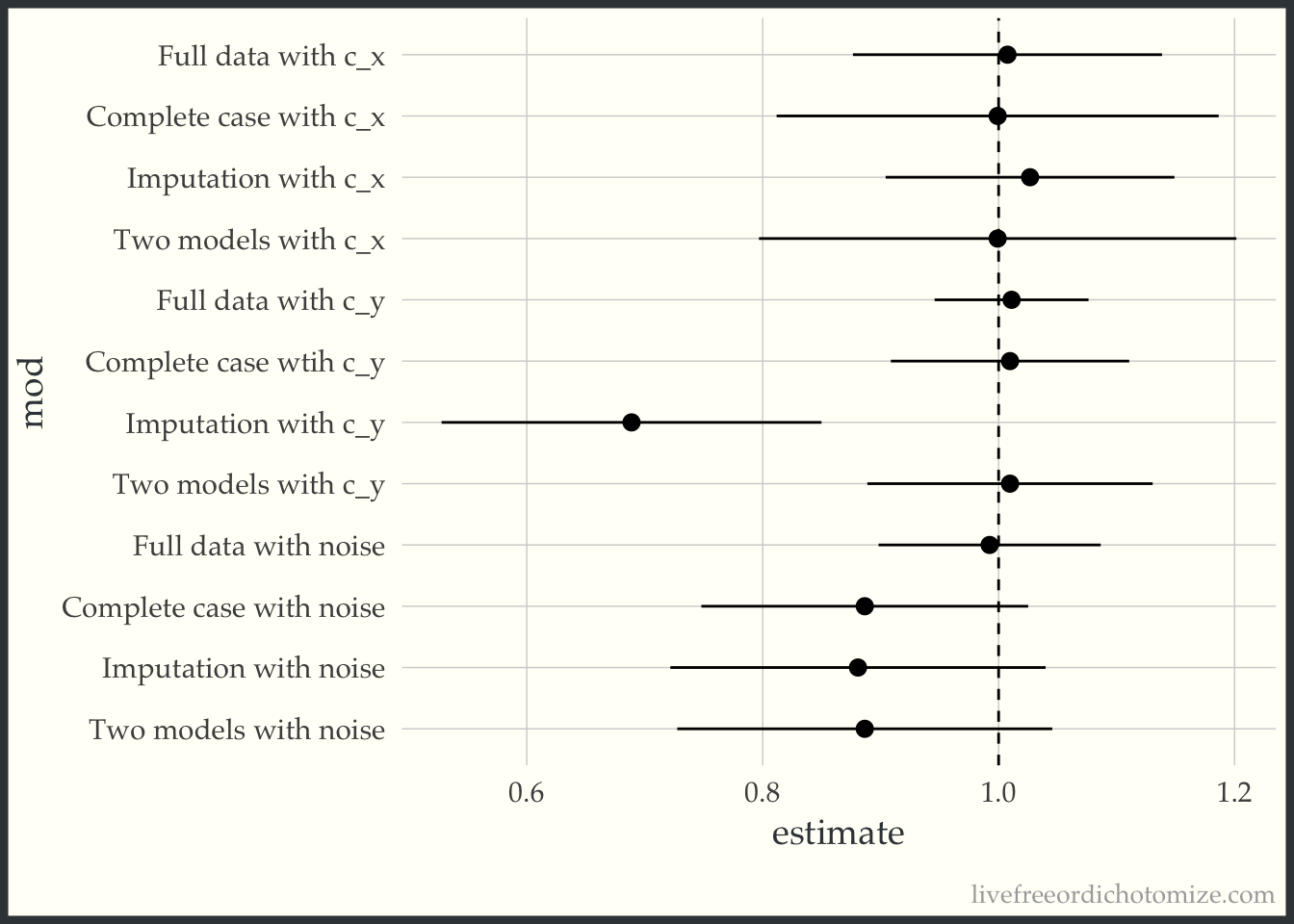So there you have it, two wrong models are rarely better than one.

In writing this post, I found that I was getting biased results when I was correctly specifying my imputation model when using the `{mice}` defaults (which is why the code above specifies `norm.predict` for the method, forcing it to use linear regression, as the data were generated). I didn’t understand why this is happening until some helpful friends on Twitter explained it (thank you Rebecca, Julian, and Mario. I’ll show you what is happening and then I’ll show a quick explanation. Let’s try to redo the imputation models using the defaults:

``````imp_default_c_x <- mice(
data,
m = 5,
formulas = list(x_obs ~ c_x),
print = FALSE)``````
``````mod_imp_c_x <- with(imp_default_c_x, lm(y ~ x_obs)) |>
pool() |>
tidy(conf.int = TRUE) |>
filter(term == "x_obs") |>
select(estimate, conf.low, conf.high)

mod_imp_c_x``````
``````   estimate  conf.low conf.high
1 0.7353276 0.6194273 0.8512279``````

``````mod_double_c_x <-  with(imp_default_c_x, lm(y ~ x_obs + c_x)) |>
pool() |>
tidy(conf.int = TRUE) |>
filter(term == "x_obs") |>
select(estimate, conf.low, conf.high)

mod_double_c_x``````
``````   estimate conf.low conf.high
1 0.4602637  0.30023 0.6202974``````

Even worse!!

``````imp_default_c_y <- mice(
data,
m = 5,
formulas = list(x_obs ~ c_y),
print = FALSE)``````
``````mod_imp_c_y <- with(imp_default_c_y, lm(y ~ x_obs)) |>
pool() |>
tidy(conf.int = TRUE) |>
filter(term == "x_obs") |>
select(estimate, conf.low, conf.high)

mod_imp_c_y``````
``````   estimate  conf.low conf.high
1 0.3405789 0.1126282 0.5685295``````

YIKES!

``````mod_double_c_y <-  with(imp_default_c_y, lm(y ~ x_obs + c_y)) |>
pool() |>
tidy(conf.int = TRUE) |>
filter(term == "x_obs") |>
select(estimate, conf.low, conf.high)

mod_double_c_y``````
``````   estimate  conf.low conf.high
1 0.5128878 0.3216547 0.7041208``````

Better since we are conditioning on `c_y` (but still bad!)

``````imp_default_noise <- mice(
data,
m = 5,
formulas = list(x_obs ~ noise),
print = FALSE) ``````
``````mod_imp_noise <-  with(imp_default_noise, lm(y ~ x_obs)) |>
pool() |>
tidy(conf.int = TRUE) |>
filter(term == "x_obs") |>
select(estimate, conf.low, conf.high)

mod_imp_noise``````
``````   estimate  conf.low conf.high
1 0.4076967 0.2352155 0.5801779``````

EEK!

``````mod_double_noise <-  with(imp_default_noise,lm(y ~ x_obs + noise)) |>
pool() |>
tidy(conf.int = TRUE) |>
filter(term == "x_obs") |>
select(estimate, conf.low, conf.high)

mod_double_noise``````
``````   estimate  conf.low conf.high
1 0.4124791 0.2497624 0.5751959``````

Let’s put those in the original plot:

``````bind_rows(
mod_full_c_x,
mod_full_c_y,
mod_full_noise,
mod_cc_c_x,
mod_cc_c_y,
mod_cc_noise,
mod_imp_c_x,
mod_imp_c_y,
mod_imp_noise,
mod_double_c_x,
mod_double_c_y,
mod_double_noise
) |>
mutate(
mod = factor(c("Full data with c_x",
"Full data with c_y",
"Full data with noise",
"Complete case with c_x",
"Complete case wtih c_y",
"Complete case with noise",
"Default Imputation with c_x",
"Default Imputation with c_y",
"Default Imputation with noise",
"Two models with c_x",
"Two models with c_y",
"Two models with noise" ),
levels = c("Full data with c_x",
"Complete case with c_x",
"Default Imputation with c_x",
"Two models with c_x",
"Full data with c_y",
"Complete case wtih c_y",
"Default Imputation with c_y",
"Two models with c_y",
"Full data with noise",
"Complete case with noise",
"Default Imputation with noise",
"Two models with noise" )),
mod = fct_rev(mod),
) -> to_plot

ggplot(to_plot, aes(x = estimate, xmin = conf.low, xmax = conf.high, y = mod)) +
geom_pointrange() +
geom_vline(xintercept = 1, lty = 2)``````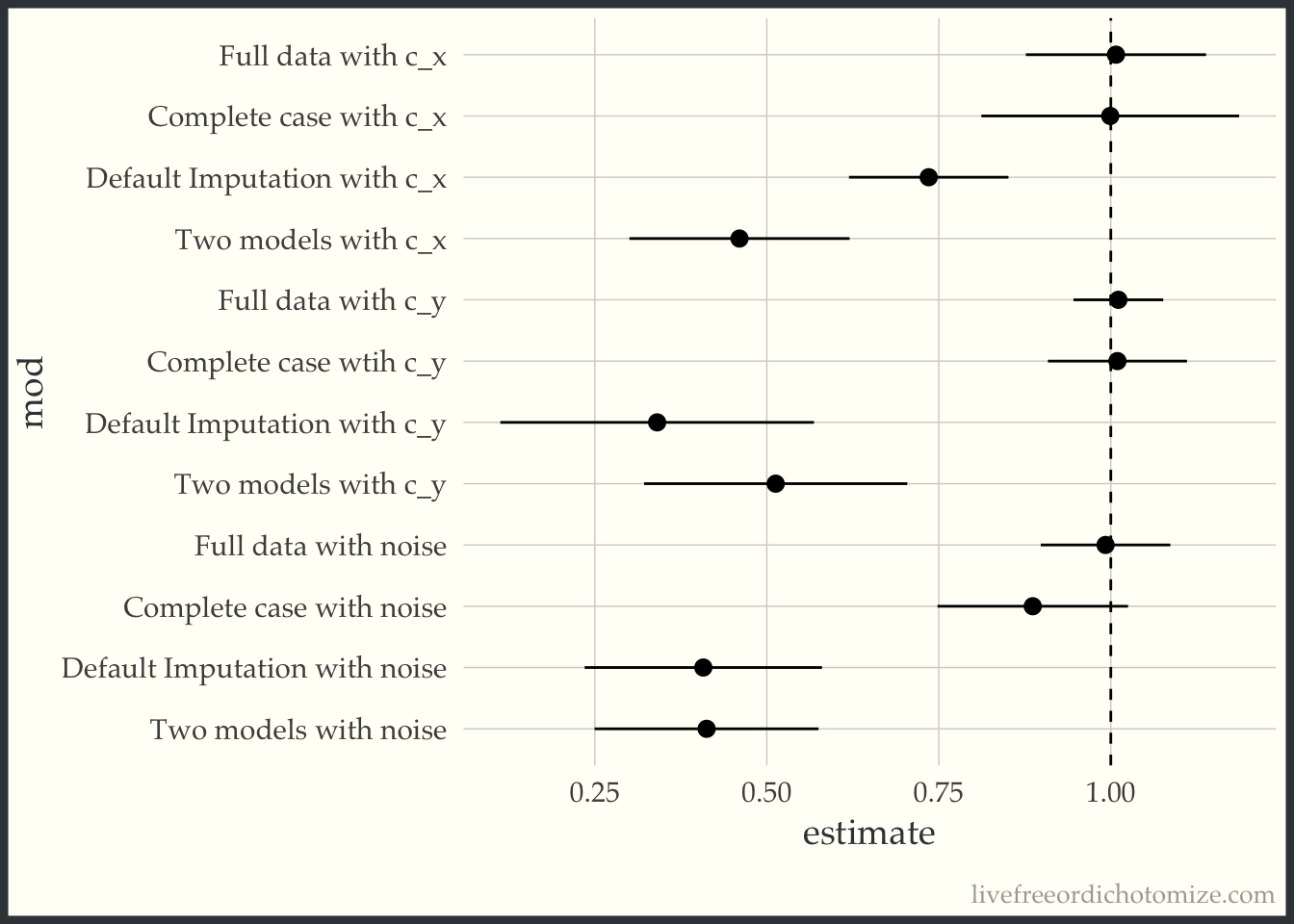AHH! This makes me so scared of imputation!!

Rebecca Andridge’s tweet finally helped me see why this is happening. The way the missing data is generated, larger values of `c_x` have a higher probability of missingness, and for particularly high values of `c_x` that probability is almost 1.

``````ggplot(data, aes(x = x, y = y, color = factor(x_miss))) +
geom_point() +
geom_vline(xintercept = 2.31, lty = 2) +
labs(color = "missing")``````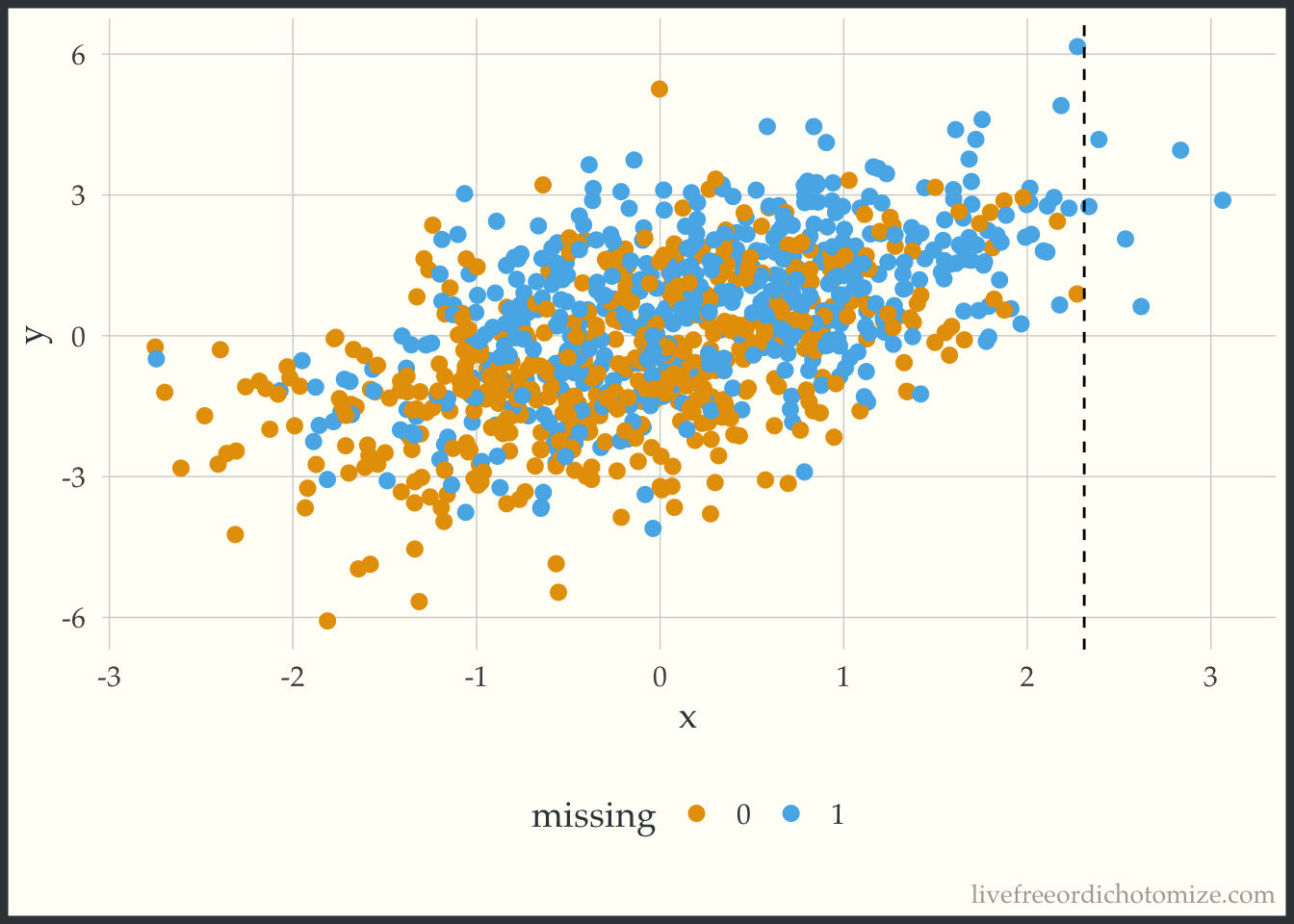Take a look at the plot above. We have no non-missing `x` values that are greater than 2.3. The way predictive mean matching (the default `{mice}` method) works is it finds the observation(s) that have the closest predicted value to the observation that is missing a data point and gives you that non-missing data point’s value. So here, we are essentially truncating our distribution at 2.3, since that is the highest value observed. Any value that would have been higher is going to be necessarily too small instead of the right value (this is different from the linear model method used in the first part of this post, which allows you to extrapolate). This is supposed to be a less biased approach, since it doesn’t allow you to extrapolate beyond the bounds of your observed data, but it can actually induce bias when you have pockets of missingness with no observed `x`s (which I would argue might happen frequently!). Here is an example of one of the imputed datasets, notice nothing is above that 2.3 line!

``````ggplot(complete(imp_default_c_x), aes(x = x_obs, y = y, color = factor(x_miss))) +
geom_point() +
scale_x_continuous(limits = c(-2.8, 3.1)) +
geom_vline(xintercept = 2.31, lty = 2) +
labs(color = "imputed") ``````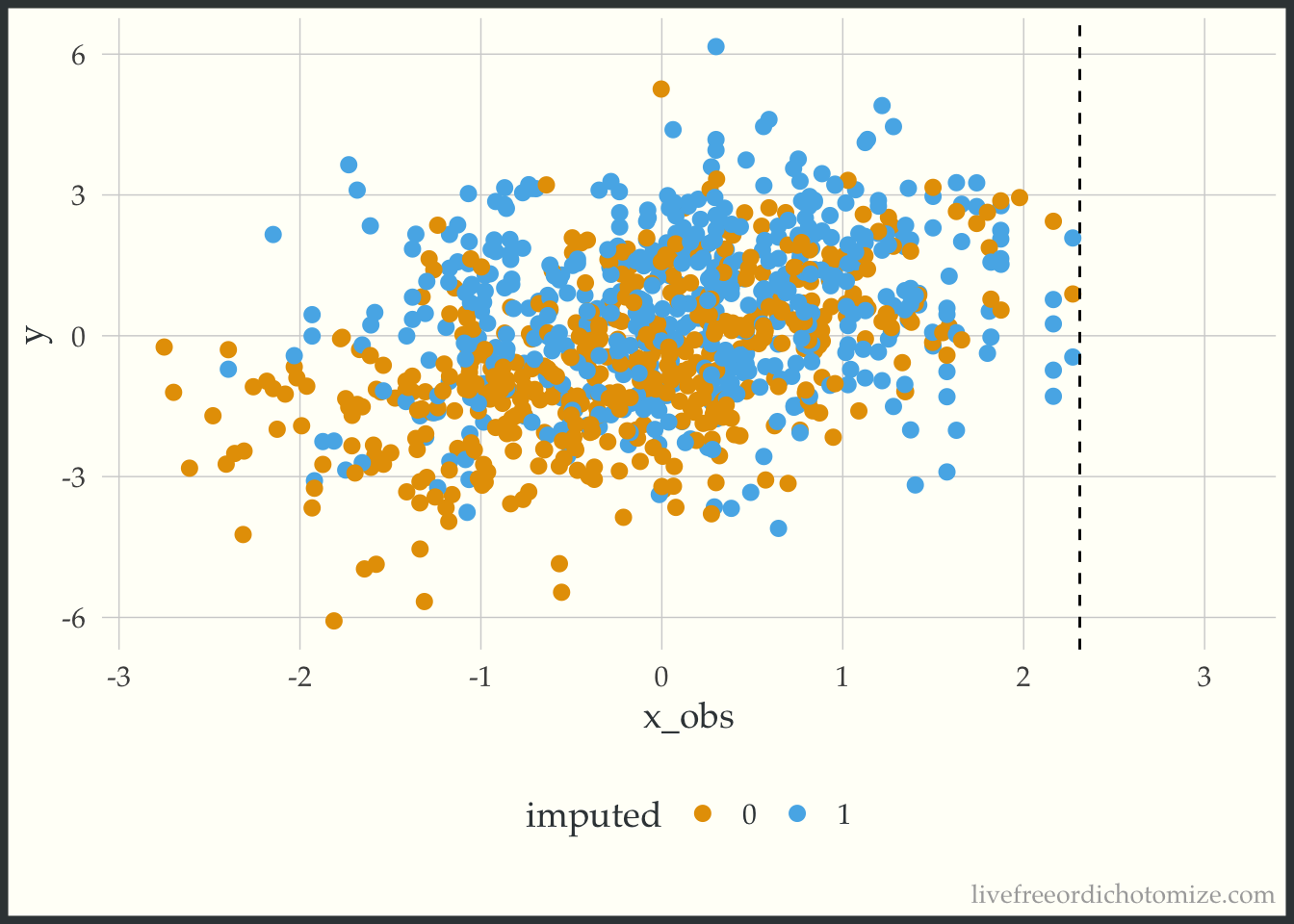## What if we use `y` in the imputation models

Including `y` in the imputation model is definitely recommended, as was hammered home for me by the wonderful Frank Harrell, but I’m not sure this recommendation has permeated through the field yet (although this paper re-iterating this result just came out yesterday so maybe it is!).

Let’s see how that improves our imputation models:

``````imp_y_c_x <- mice(
data,
m = 5,
formulas = list(x_obs ~ c_x + y),
print = FALSE)``````
``````mod_imp_c_x <- with(imp_y_c_x, lm(y ~ x_obs)) |>
pool() |>
tidy(conf.int = TRUE) |>
filter(term == "x_obs") |>
select(estimate, conf.low, conf.high)

mod_imp_c_x``````
``````  estimate  conf.low conf.high
1 1.034138 0.9109341  1.157343``````

Beautiful!

``````mod_double_c_x <-  with(imp_y_c_x, lm(y ~ x_obs + c_x)) |>
pool() |>
tidy(conf.int = TRUE) |>
filter(term == "x_obs") |>
select(estimate, conf.low, conf.high)

mod_double_c_x``````
``````  estimate  conf.low conf.high
1  1.08074 0.8772936  1.284186``````

A bit worse, but not bad!

``````imp_y_c_y <- mice(
data,
m = 5,
formulas = list(x_obs ~ c_y + y),
print = FALSE)``````
``````mod_imp_c_y <- with(imp_y_c_y, lm(y ~ x_obs)) |>
pool() |>
tidy(conf.int = TRUE) |>
filter(term == "x_obs") |>
select(estimate, conf.low, conf.high)

mod_imp_c_y``````
``````   estimate  conf.low conf.high
1 0.9298631 0.8137157  1.046011``````

Not bad!! A bit biased but way better.

``````mod_double_c_y <-  with(imp_y_c_y, lm(y ~ x_obs + c_y)) |>
pool() |>
tidy(conf.int = TRUE) |>
filter(term == "x_obs") |>
select(estimate, conf.low, conf.high)

mod_double_c_y``````
``````  estimate  conf.low conf.high
1  1.01812 0.9415964  1.094644``````

Love it, looks great after conditioning on `c_y`

``````imp_y_noise <- mice(
data,
m = 5,
formulas = list(x_obs ~ noise + y),
print = FALSE) ``````
``````mod_imp_noise <-  with(imp_y_noise, lm(y ~ x_obs)) |>
pool() |>
tidy(conf.int = TRUE) |>
filter(term == "x_obs") |>
select(estimate, conf.low, conf.high)

mod_imp_noise``````
``````   estimate  conf.low conf.high
1 0.9671593 0.8580147  1.076304``````

Oo lala, even does well when we don’t have the right model (this makes sense because we are using `y`!)

``````mod_double_noise <-  with(imp_y_noise, lm(y ~ x_obs + noise)) |>
pool() |>
tidy(conf.int = TRUE) |>
filter(term == "x_obs") |>
select(estimate, conf.low, conf.high)

mod_double_noise``````
``````   estimate  conf.low conf.high
1 0.9697639 0.8612499  1.078278``````

Let’s put those in the original plot:

``````bind_rows(
mod_full_c_x,
mod_full_c_y,
mod_full_noise,
mod_cc_c_x,
mod_cc_c_y,
mod_cc_noise,
mod_imp_c_x,
mod_imp_c_y,
mod_imp_noise,
mod_double_c_x,
mod_double_c_y,
mod_double_noise
) |>
mutate(
mod = factor(c("Full data with c_x",
"Full data with c_y",
"Full data with noise",
"Complete case with c_x",
"Complete case wtih c_y",
"Complete case with noise",
"Imputation with c_x and y",
"Imputation with c_y and y",
"Imputation with noise and y",
"Two models with c_x",
"Two models with c_y",
"Two models with noise" ),
levels = c("Full data with c_x",
"Complete case with c_x",
"Imputation with c_x and y",
"Two models with c_x",
"Full data with c_y",
"Complete case wtih c_y",
"Imputation with c_y and y",
"Two models with c_y",
"Full data with noise",
"Complete case with noise",
"Imputation with noise and y",
"Two models with noise" )),
mod = fct_rev(mod),
) -> to_plot

ggplot(to_plot, aes(x = estimate, xmin = conf.low, xmax = conf.high, y = mod)) +
geom_pointrange() +
geom_vline(xintercept = 1, lty = 2)``````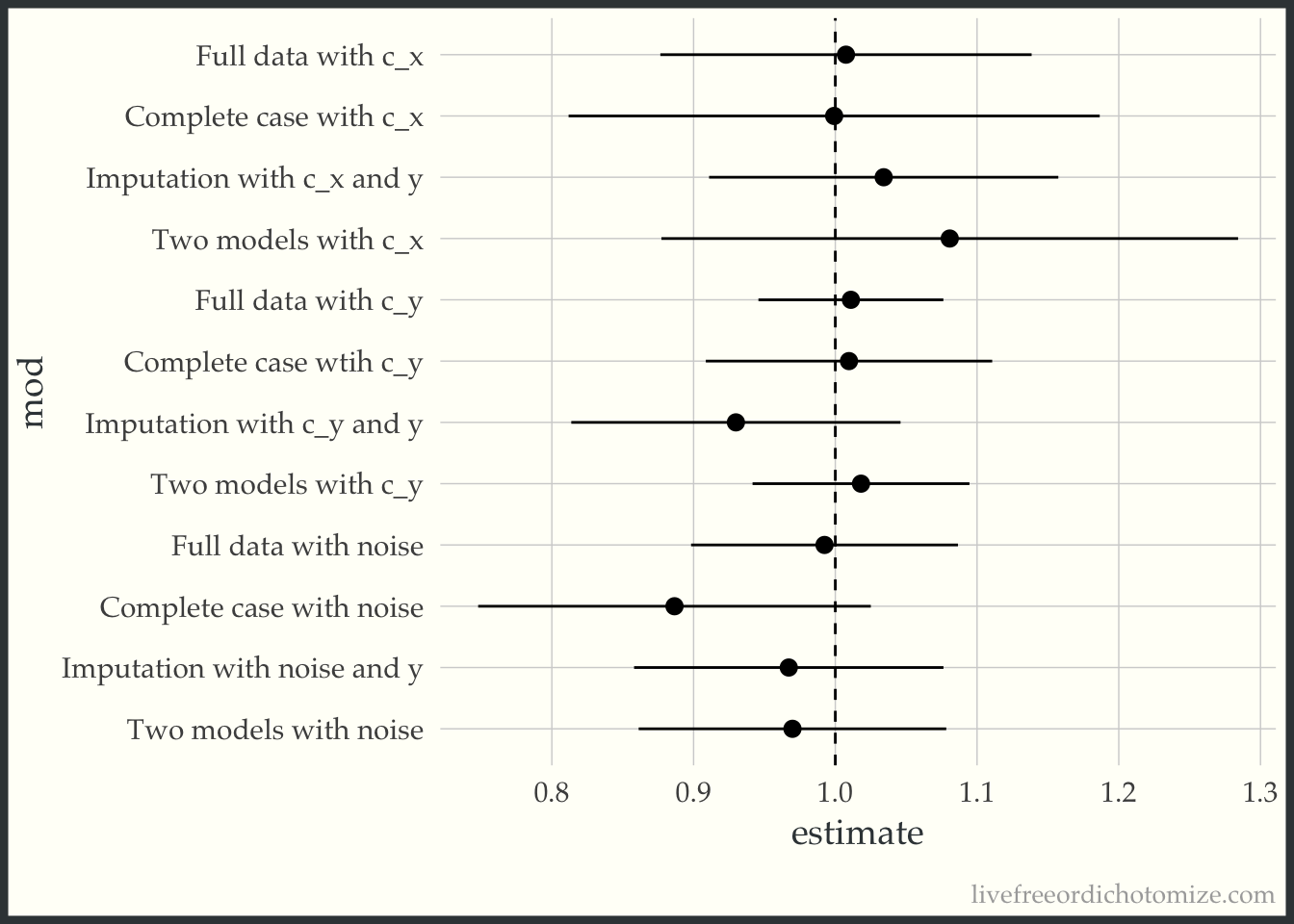Pretty good! Maybe I’m feeling a little better about imputation. Maybe. But it had better include the outcome (which I’ll admit feels very weird for my little causal brain).